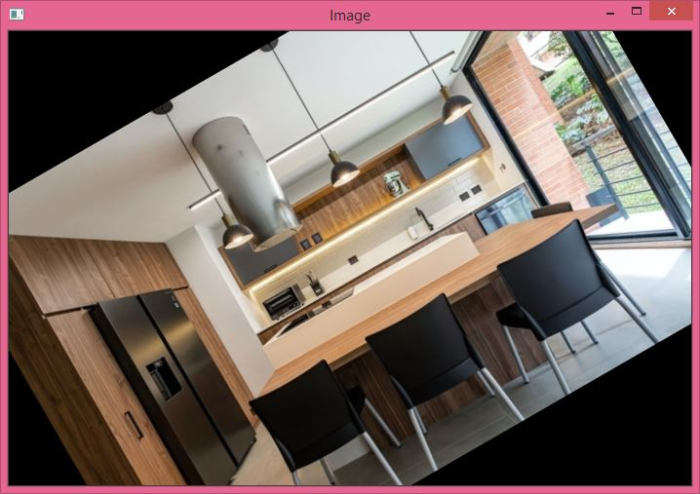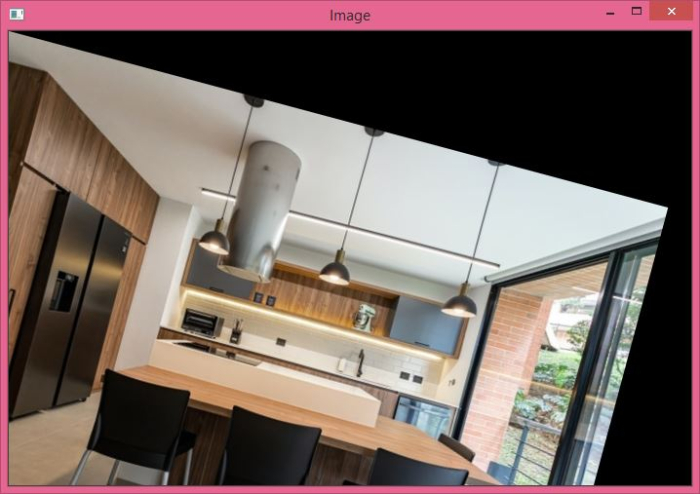# How to perform image rotation in OpenCV using Python?

To perform image rotation by an angle, we first need to get the rotation matrix. To find the rotation matrix, we apply cv2.getRotationMatrix2D() function. The syntax for this function is −

M = cv2.getRotationMatrix2D(cr, degree, scale)


Where cr is the center of rotation, degree is the angle by which image is rotated and scale is scale factor to scale up or scale down the image.

The rotation matrix M is a 2×2 matrix (numpy array). We pass the rotation matrix M to cv2.warpAffine() function as an argument. See the syntax below −

## Syntax

cv2.warpAffine(img, M, (width, height))


Here,

• img − The input image which is to be rotated.

• M − Rotation matrix defined above.

• (width, height) − Width and height of the image after rotation.

## Steps

To perform an image rotation, you can follow the steps given below −

Import the required library. In all the following Python examples, the required Python library is OpenCV. Make sure you have already installed it.

import cv2


Read the input image using cv2.imread() function. Pass the full path of the input image.

img = cv2.imread('interior1.jpg')


Define a rotation matrix M using cv2.getRotationMatrix2D(cr, degree, scale) function. Pass the center of rotation cr, rotation angle degree, and scale factor scale to the function, for example, cr=(width/2, height/2), degree=30, scale=1.

M = cv2.getRotationMatrix2D(cr,30,1)


Rotate the image using cv2.warpAffine() method.

img = cv2.warpAffine(img,M,(w,h))


Display the rotated image.

cv2.imshow('Image Translation', img)
cv2.waitKey(0)
cv2.destroyAllWindows()


Let's look at some examples for a clear understanding about the question.

## Input Image

We use the following image as the input file in the examples below.## Example 1

In this program, we rotate the input image by an angle of 30 degrees in anticlockwise direction. The center of rotation is the midpoint (center) of the image, i.e., (width/2, height/2).

# import required libraries
import cv2

# access height and width of the image
height, width, _ = img.shape

# define center of rotation
cr = (width/2,height/2)

# get the rotation matrix
M = cv2.getRotationMatrix2D(cr,30,1)

# apply warpAffine() method to perform image rotation
dst = cv2.warpAffine(img,M,(width,height))

# display the rotated image
cv2.imshow('Image',dst)
cv2.waitKey(0)
cv2.destroyAllWindows()


## Output

On execution, it will produce the following output window −Notice that the mean squared error is a scalar value.

## Example 2

In this program, we rotate the input image by an angle of 15 degrees in clockwise direction. The center of rotation is (0,0).

import cv2
import numpy as np

h,w, _ = img.shape

M = cv2.getRotationMatrix2D((0,0),-15,1)
dst = cv2.warpAffine(img,M,(w,h))

cv2.imshow('Image',dst)
cv2.waitKey(0)
cv2.destroyAllWindows()


## Output

When you run the above Python program, it will produce the following output window −